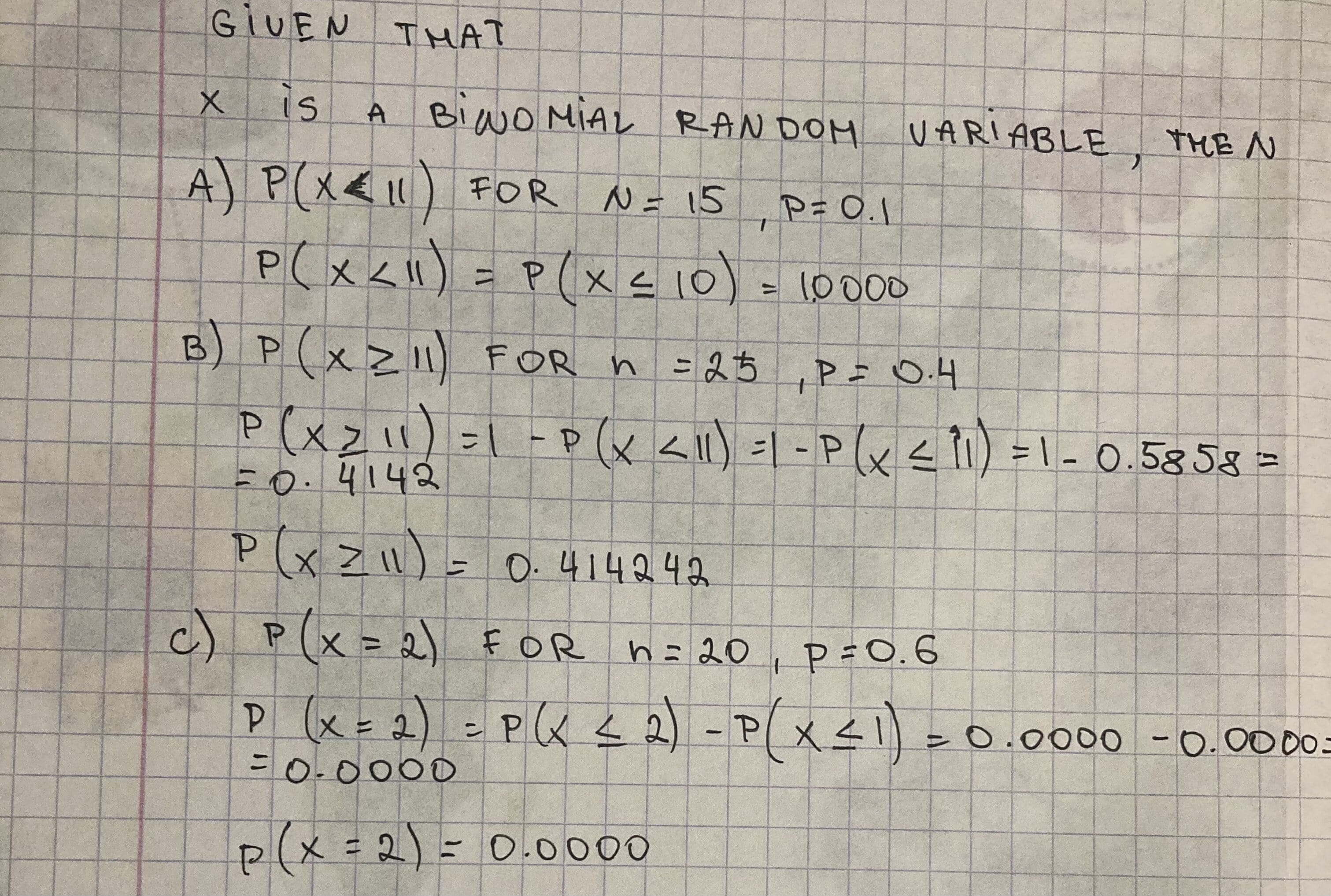If x is a binomial random variable, find the probabilities below by using the binomial probability table.emancipezN 2020-11-22 Answered

If x is a binomial random variable, find the probabilities below by using the binomial probability table.

a) $$P(x < 11)\ for\ n = 15, p = 0.1$$

b) $$P(x \geq 11)\ for\ n = 25, p = 0.4$$

c) $$P(x = 2)\ for\ n = 20, p = 0.6$$

• Questions are typically answered in as fast as 30 minutes

Solve your problem for the price of one coffee

• Math expert for every subject
• Pay only if we can solve itTasneem Almond.